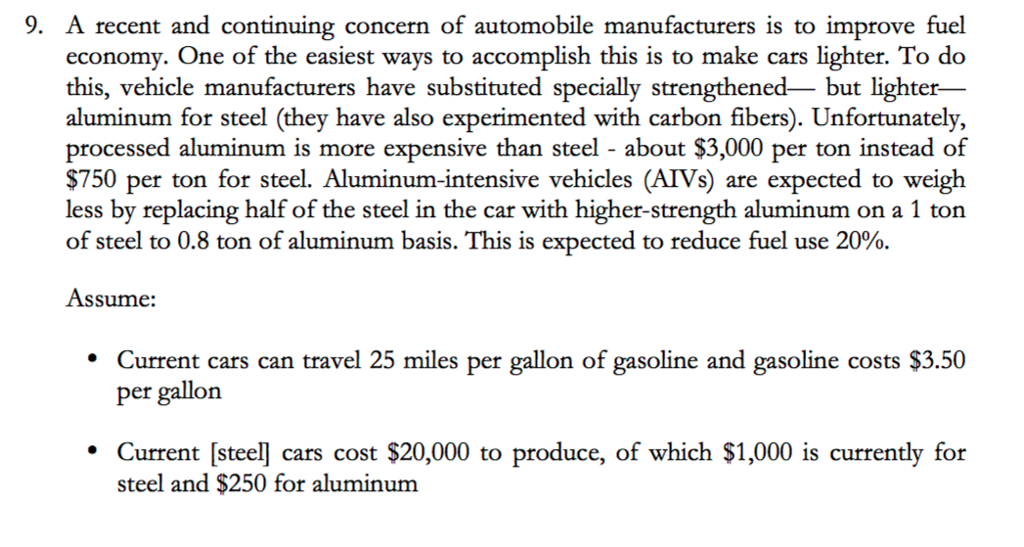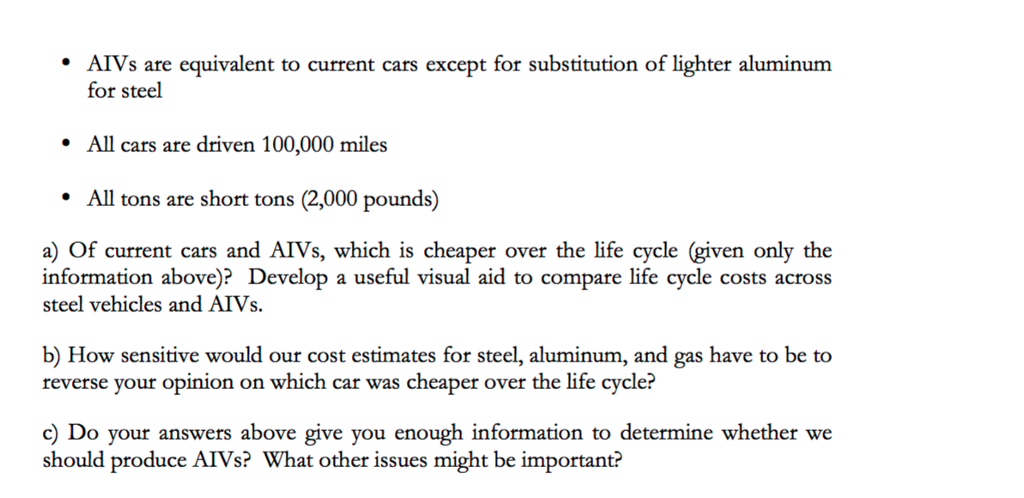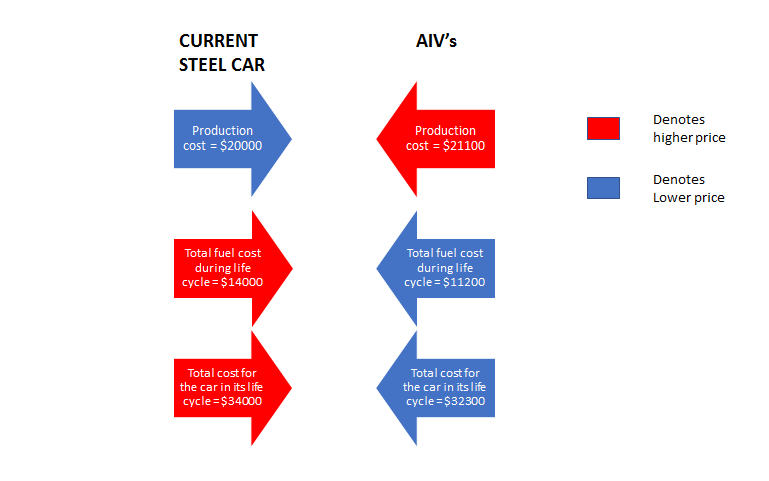# Question & Answer: A recent and continuing concern of automobile manufacturers is to improve fuel…..A recent and continuing concern of automobile manufacturers is to improve fuel economy. One of the easiest ways to accomplish this is to make cars lighter. To do this, vehicle manufacturers have substituted specially strengthened- but lighter- aluminum for steel (they have also experimented with carbon fibers). Unfortunately, processed aluminum is more expensive than steel about \$3,000 per ton instead of \$750 per ton for steel. Aluminum-intensive vehicles (AIVs) are expected to weigh less by replacing half of the steel in the car with higher-strength aluminum on a 1 ton of steel to 0.8 ton of aluminum basis. This is expected to reduce fuel use 20%. Assume: Current cars can travel 25 miles per gallon of gasoline and gasoline costs \$3.50 per gallon Current [steel] cars cost \$20,000 to produce, of which \$1,000 is currently for steel and \$250 for aluminum AIVs are equivalent to current cars except for substitution of lighter aluminum for steel All cars are driven 100,000 miles All tons are short tons (2,000 pounds) a) Of current cars and AIVs, which is cheaper over the life cycle (given only the information above)? Develop a useful visual aid to compare life cycle costs across steel vehicles and AIVs. b) How sensitive would our cost estimates for steel, aluminum, and gas have to be to reverse your opinion on which car was cheaper over the life cycle? c) Do your answers above give you enough information to determine whether we should produce AIVs? What other issues might be important?

(a)

Don't use plagiarized sources. Get Your Custom Essay on
Question & Answer: A recent and continuing concern of automobile manufacturers is to improve fuel…..
GET AN ESSAY WRITTEN FOR YOU FROM AS LOW AS \$13/PAGE

Given,

Fuel price = \$3.50 per gallon

Total travel distance of the car = 100000 miles

Price of steel = \$750 per ton

Price of aluminium = \$ 3000 per ton

Production cost of current steel car = \$20000

Current Steel Car,

Amount Spent for steel = \$1000

Amount Spent for Aluminium = \$250

Weight of steel used = (Total Amount Spent for steel) / (cost of steel per ton) = 1000/750

= 4/3 tons = 1.3333 tons = 1.3333 * (2000) pounds = 2666.667 pounds

Weight of aluminium used = (Total amount spent for aluminium) / (cost of aluminium per ton)

= 250/3000 = 1/12 tons = 0.083333 tons = 0.08333 * (2000) pounds

= 166.6667 pounds

Total weight of the curent steel car (considering only the steel and aluminium parts) = 1.3333 + 0.08333

= 1.41667 tons

= 2833.34 pounds

Mileage of the current steel car = 25 miles per gallon

Fuel consumption of the car during its life cycle = (total distance traveled during its life time) / ( mileage of the car ) = 100000 miles / 25 = 4000 gallons

Price of fuel consumed = 4000 gallons * ( per gallon price of gasoline) = 4000 * \$3.50 = \$14000

Total expenditure on the car during its life cycle = production cost + fuel cost = \$20000 + \$14000

\$34000

For New Aluminium Intensive Vehicle (AIV’s),

Amount of steel used is half of the current one = (4/3 ) tons divide by 2 = 2/3 tons = 0.6667 tons

Cost for the total steel used = 2/3 * \$750 = \$500

Amount of aluminium used = already used aluminium + half of the steel replaced with aluminium

= 0.08333 + (2/3) * 0.8 = 0.61667 tons

Cost of the aluminium used = 0.61667 * 3000 = \$1850

Total production cost of car ( assuming all other production cost remains same except material price)

= (current steel car production cost – cost of materials) + (cost of the new material composition )

= (20000 – 1250) + (1850 + 500) = \$21100

Increase in mileage = 20% reduction in fuel usage = 25 miles per 0.8 gallon = 25/ 0.8 miles per gallon

= 31.25 miles per gallon

Total fuel cost to travel 100000 miles = (100000 / 31.25 ) * fuel price per gallon = (100000 / 31.25 ) * 3.50 = \$11200

Total cost of the AIV’s during its life cycle = production cost + travel cost = 21100 + 11200 = \$32300

Therefore, minimum cost over the life time is for AIV’s(b)

Considering the sensitivity of each individually,

For aluminium,

contribution of aluminium in the AIV’s = 0.61667 tons =\$1850

To equate the total life time cost of both AIV’s (\$32300) and steel car(\$34000) = 34000 – 32300 = \$1700 should be higher

Therefore increase in Aluminium price required = (1850 + 1700) / 0.61667 = \$5757 per ton ( any cost higher would reverse our previous answer)

For steel, a reduction in the price of the steel would render the decision in the reverse direction.

Similarly for fuel also, reduction in fuel price would equate the two values and thus change our decision.

(c)

No, the answers above is not enough to determine whether to produce AIV’s.

Other issues are as follows:

1, Availability of the aluminium.

2, Transit cost for Aluminium from supplier to the manufacturer.

3, Holding cost of the Aluminium.

4, Increase in cost of other production related activities with the introduction of aluminium.